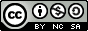## Saturday, June 14, 2008

### Shell Script To Produce Prime Numbers On Linux And Unix

Hey There,

You might recall (quite a while back) the last time we put out a Linux or Unix script involving a somewhat-complex mathematical formula. The most recent post had to deal with factorial generation and the only other mostly-math post we did prior to that was about generating Fibonacci numbers up to any user-defined limit.

Today we're going to look at a similar issue, and develop a similar script. This time we'll work on something that, on its face, seems a bit easier to figure out. Today we're going to create a script that will list out all "prime" numbers up to a user-defined limit. We'll stay away from "relative primes" since (by definition) any two numbers, that aren't primes, share a greatest common factor (Sometimes referred to as the lowest common denominator in fractions) of 1. So non-prime numbers like 9 (1 x 3 x 9) and 25 (1 x 5 x 25) are considered relative primes. The definition basically applies to any numbers that aren't primes. You could easily derive those just by removing all the prime numbers from the positive integer set you define when you invoke the script, which can be easily done, like this:

host # ./primes.sh 100

Composite numbers are the result of the multiplication of prime numbers. We'll take a look at scripting out composites for our next post. It should be a natural extension of today's script.

It's interesting to note, though, that the number 1 is not considered a prime, because it only has one factor: itself. All other numbers, that are available for prime status, have two factors: themselves and themselves divided by 1 (with the result being a positive integer). Of course, the number 1 divided by 1 is zero; therefore, it only has one factor and it's not a prime. It is also not considered a composite. We'll be avoiding the dreaded zero today, as well, since it's neither a prime nor a composite either.

With all that muck out of the way (I hope ;), let's take a look at defining prime numbers on a formula-based scale. There is an excellent reference online at mathforum.org that goes into detail about many different ways (some better, some worse) to go about deriving primes to the nth degree. For our purposes, we're going to dispense with the Greek alphabet and look at solving the problem as purely as possible, even though that might not be the "most efficient" way to do it. Sometimes (especially if you're not a math wizard, like me), understanding how to generate numbers that meet certain criteria, is more important than applying advanced mathematical formulae which, for all I know, may or may not even be completely valid. If you're ever up for twisting your brain into a knot, try to absolutely prove any advanced mathematical formula. For kicks, ask an advanced mathemetician to do the same ;)

For those of you who are curious (since there are so many theorems out there), our script today is based on Wilson's Theorem, which basically states:

An integer p > 1 is prime if and only if the factorial (p - 1)! + 1 is divisible by p

In other words, if you take your chosen number, subtract 1 from that, get the factorial value (which we've gone through in more detail in our post regarding factorial generation) of the resulting subtraction and then add 1 to it, the number is only prime if the modulus (or remainder) of that resulting number's division by the original number is equal to 0 (or, depending on how you prefer to think of it, if the resulting number is absolutely divisible by the original number).

I hope you enjoy this script. I wangled the factorial function so that it's quite a bit faster than the "bash" method shown in our factorial generation post from earlier on. In fact, here's a look at the time savings from Linux running on a now-obsolete PC :)

`host # time ./primes.sh.bak 35Generating Prime Numbers Up To 352 3 5 7 11 13 17 19 35All Set!real    3m21.994suser    0m18.445ssys     0m40.857shost # time ./primes.sh 35Generating Prime Numbers Up To 352 3 5 7 11 13 17 19 35All Set!real    0m8.173suser    0m1.464ssys     0m2.407s`

Note that this script can't find the highest prime number (I don't think anyone's figured that out yet), but it can figure out the highest prime number your system is capable of calculating. Close enough ;)

Cheers,`#!/bin/bash## primes.sh - find all prime numbers up to a certain number# 2008 - Mike Golvach - eggi@comcast.net## Creative Commons Attribution-Noncommercial-Share Alike 3.0 United States License#factorial ()  { local factorial_count=\$1 if [ "\$factorial_count" -eq 0 ] then  factorial_count=1 fi for (( factor=\$((factorial_count -1)); \$factor >= 1; --factor )) do  factorial_count=\$((\$factorial_count * \$factor)) done echo \$factorial_count}prime_number ()  { local prime=\$1 p_minus_1=\$((\$prime - 1)) fact_p_minus_1=`factorial "\$p_minus_1"` fact_plus_1=\$((\$fact_p_minus_1 + 1)) echo \$fact_plus_1}highest_number=\$1if [ -z \$highest_number ]then echo echo "Usage: \$0 highestNumber" echo exit 1fiif [ \$highest_number -eq 0 ]then echo echo "Sorry.  0 is not a prime number" echo exit 0elif [ \$highest_number -eq 1 ]then echo echo "Sorry.  0 and 1 are not prime numbers" echo exit 0fiecho "Generating Prime Numbers Up To \$highest_number"if [ \$highest_number -eq 2 ]then echo echo -n "2" else echo echo -n "2 3 " ficount=4while [ \$count -le \$highest_number ]do prime_return=`prime_number "\$count"` prime_test=\$((\$prime_return % count)) if [ \$prime_test -eq 0 ] then  echo -n "\$count " fi count=\$((\$count + 1))doneechoechoecho "All Set!"echoexit 0`